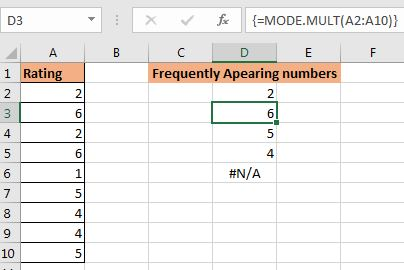# How to Use Excel MODE.MULT function

The Excel MODE.MULT function was introduced in Excel 2010 along with MODE.SNGL function. Prior to that excel only had MODE function that returned the most frequent number in a range or array.

The problem with the MODE function is that it returns first value that has occurred most of the time. If there are two numbers that have occurred thrice then it would return only first number. The MODE.MULT function returns multiple values.

Syntax of MODE.MULT function:

 {=MODE.MULT(numbers)}

numbers: the numbers from which you want to get your frequent numbers. It can be an array or verticle range.

Let's have a quick example.

Example:

Here I have a data set that has duplicate numbers. I want to get all frequently appearing numbers from this range.In range A2:A10 we have some numbers. Let us use the MODE.MULT function to get all frequently appearing numbers.

Select a vertical range where you want your output. I have selected D2:D6. Start writing your formula and hit CTRL+SHIFT+ENTER drag down.

 {=MODE.MULT(A2:A10)}

This fills the range D2:D6 with most frequently appeared numbers from range A2:A10. The frequency if these numbers are equal. You can see last as #N/A. It means there's no other cell with the same number of frequency.Important Notes:

• The MODE.MULT function returns all numbers with the top frequency. For example, top frequency is 3, then MODE.MULT function will return all values with frequency 3. In our example, the top frequency was 2, hence function returned all numbers with the frequency of 2, which are 2,6,5, and 4.
• It is a multicell array formula. It means you have to select the range where you want your output and then start writing the formula. After writing the formula, you have to hit CTRL+SHIFT+ENTER.
• If the range or array does not contain any duplicate number then the function will return a #N/A error.
• If you select a range more than the required, extra cells will be filled with #N/A error.

So yeah guys, this how you use MODE.MULT function in excel. I hope I was explanatory enough. If you have any doubts regarding this function or any other function in excel, feel free to ask in the comments section below.

Related Articles:

How to Use the MODE function | Most frequently appearing numbers can be calculated using the MODE function of Excel.

Get most frequently appearing text in Excel | Learn how to get frequently appearing text in excel.

Lookup Frequently Appearing Text with Criteria in Excel | To get the most frequently appearing text in excel with criteria you just need to put an if condition before match function.

Retrieving the First Value in a List that is Greater / Smaller than a Specified Value | Learn how to lookup first value that is greater than a specified value. The generic formula to lookup is...

How to retrieve the entire row of a matched value| It is easy to look up and retrieve the entire row from a table. Let's see how can we do this lookup.

How to Retrieve Latest Price in Excel | To look up the latest price from a range you must have your data sorted. Once sorted use this formula...

Popular Articles:

50 Excel Shortcuts to Increase Your Productivity | Get faster at your task. These 50 shortcuts will make you work even faster on Excel.

How to use the VLOOKUP Function in Excel | This is one of the most used and popular functions of excel that is used to lookup value from different ranges and sheets.

How to use the COUNTIF function in Excel 2016 | Count values with conditions using this amazing function. You don't need to filter your data to count specific values. Countif function is essential to prepare your dashboard.

How to use the SUMIF Function in Excel | This is another dashboard essential function. This helps you sum up values on specific conditions.

Terms and Conditions of use

The applications/code on this site are distributed as is and without warranties or liability. In no event shall the owner of the copyrights, or the authors of the applications/code be liable for any loss of profit, any problems or any damage resulting from the use or evaluation of the applications/code.